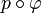# Covering map

Jump to: navigation, search

## Definition

### Uniform fiber definition

A continuous map$p:E \to B$ of topological spaces is termed a covering map with fiber equal to a discrete space$F$ if$p$ is surjective and satisfies the following condition: For every$b \in B$, there exists an open subset$U$ of$B$ containing$b$ and a homeomorphism$\varphi:U \times F \to p^{-1}(U)$ such that$p \circ \varphi$ is the projection onto the first coordinate.

In other words, a covering map is a fiber bundle with discrete fiber.

### Definition with possibly non-uniform bundle

A continuous map$p:E \to B$ of topological spaces is termed a covering map if$p$ is surjective and satisfies the following condition: For every$b \in B$, there exists an open subset$U$ of$B$ containing$b$, a discrete space$F$ (dependent upon$b$), and a homeomorphism$\varphi:U \times F \to p^{-1}(U)$ such that$p \circ \varphi$ is the projection onto the first coordinate.

The main difference between these two definitions is that in the latter definition, the discrete fiber could differ from point to point. Note that when the base space$B$ is a connected space, then the fibers at all points are homeomorphic, hence there is no conflict between the two definitions. Since covering maps are typically studied in contexts where the base space is a path-connected space, there is no ambiguity in these cases.

### Non-surjective variant

Some variants use the term covering map for a covering map onto an open subset. In other words,$p:E \to B$ is termed a covering map in this looser sense if$p$ is continuous,$p(E)$ is an open subset$V$ of$B$, and$p$ is a covering map (in the strict sense) when viewed as a map from$E$ to$V$.

### Terminology

• The space$E$ is termed the covering space.
• The space$B$ is termed the base space.
• The cardinality of the fiber$F$ is termed the degree of the covering map (this makes sense if we use the first definition, where all fibers must be homeomorphic). Note that since$F$ is a discrete space, its homeomorphism type is determined by its cardinality.

## Relation with other properties

### Stronger properties

Property Meaning Proof of implication Proof of strictness (reverse implication failure) Intermediate notions
homeomorphism continuous bijective map with continuous inverse; or a covering map of degree one |FULL LIST, MORE INFO
regular covering map covering map whose automorphism group is transitive on each fiber |FULL LIST, MORE INFO

### Weaker properties

Property Meaning Proof of implication Proof of strictness (reverse implication failure) Intermediate notions
local homeomorphism every point in the domain has an open neighborhood such that the restriction of the map to that open neighborhood is a homeomorphism covering map implies local homeomorphism |FULL LIST, MORE INFO
open map image of every open subset is open (via local homeomorphism) Local homeomorphism|FULL LIST, MORE INFO
quotient map Open map|FULL LIST, MORE INFO